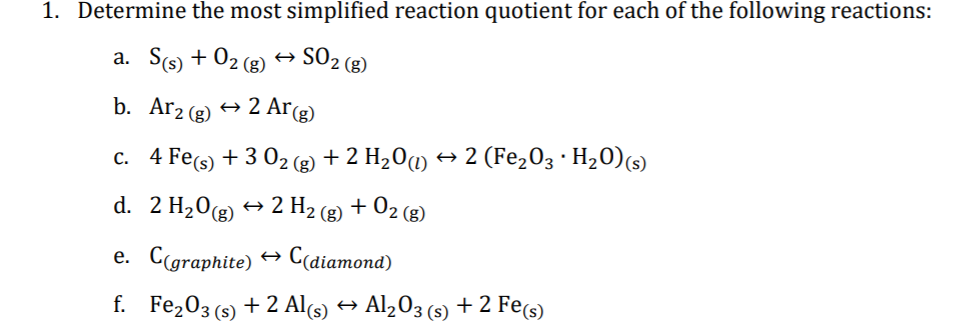# 1. Determine the most simplified reaction quotient for each of the following reactions: a. S(s) + 02 (g) SO2 (8) b. Ar2 (g) 2 Ar(g) c. 4 Fe(s) + 3 02 (2 + 2 H20m → 2 (Fe,03 · H20)(s) + 2 H2 (g) + 02 (g) d. 2 H20g) e. Cgraphite) → C(diamond) f. Fe203 (s) + 2 Al(s) → Al,03 (s) + 2 Fe(s)

Question

chemical activity questionhelp_outlineImage Transcriptionclose1. Determine the most simplified reaction quotient for each of the following reactions: a. S(s) + 02 (g) SO2 (8) b. Ar2 (g) 2 Ar(g) c. 4 Fe(s) + 3 02 (2 + 2 H20m → 2 (Fe,03 · H20)(s) + 2 H2 (g) + 02 (g) d. 2 H20g) e. Cgraphite) → C(diamond) f. Fe203 (s) + 2 Al(s) → Al,03 (s) + 2 Fe(s) fullscreen

### Want to see this answer and more?

Experts are waiting 24/7 to provide step-by-step solutions in as fast as 30 minutes!*

*Response times vary by subject and question complexity. Median response time is 34 minutes and may be longer for new subjects.
Tagged in
ScienceChemistry

### Equilibrium Concepts